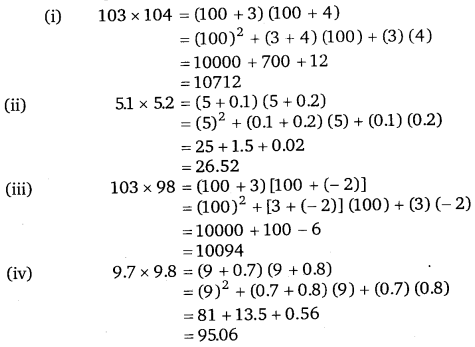# Class 8 Maths NCERT Solutions for Chapter – 9 Algebraic Expressions and Identities Ex – 9.5

### Algebraic Expressions and Identities

Question 1.
Use a suitable identity to get each of the following products,
(i)
(x + 3) (x + 3)
(ii) (2y + 5)(2y + 5)
(iii) (2a – 7) (2a – 7)
(iv)$\left( 3a-\frac { 1 }{ 2 } \right) \left( 3a-\frac { 1 }{ 2 } \right)$
(v) (1.1m – 0.4) (1.1m + 0.4)
(vi) (a2 + b2) (- a2 + b2)
(vii) (6x – 7) (6x + 7)
(viii) (- a + c) (- a + c)
(ix)$\left( \frac { x }{ 2 } +\frac { 3y }{ 4 } \right) \left( \frac { x }{ 2 } +\frac { 3y }{ 4 } \right)$
(x) (7a – 9b)(7a – 9b)

Solution: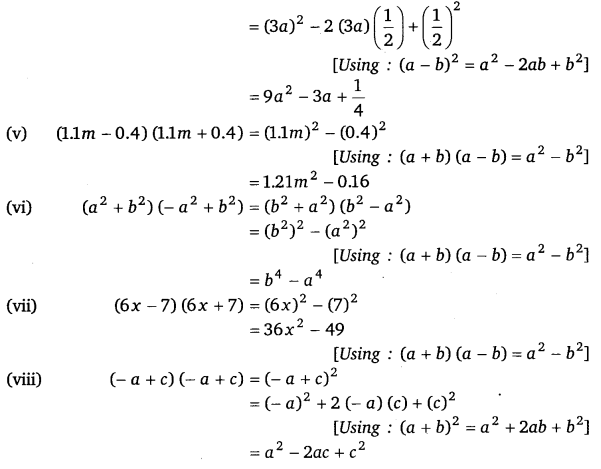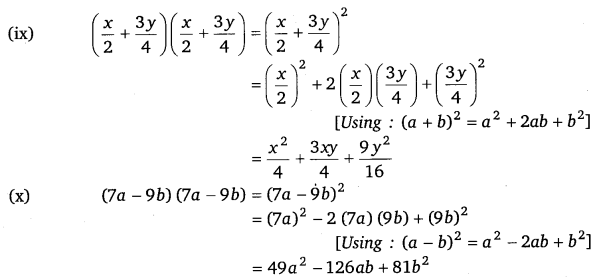Question 2.
Use the identity (x + a) (x + b) = x2 + (a + b)x + ab to find the following products.
(i)
(x + 3) (x + 7)
(ii) (4x + 5) (4x + 1)
(iii) (42 – 5) (4x – 1)
(iv) (4x + 5) (42 – 1)
(v) (2x + 5y) (2x + 3y)
(vi) (2a2 + 9) (2a2 + 5)
(vii) (xyz – 4) (xyz – 2)

Solution:Question 3.
Find the following squares by using the identities :
(i)
(b – 7)2
(ii) (xy + 3z)2
(iii) (6x2 – 5y2)
(iv)$\left( \frac { 2 }{ 3 } m+\frac { 3 }{ 2 } n \right) ^{ 2 }$
(v) (0.4p – 0.5q)2
(vi) (2xy + 5y)2

Solution: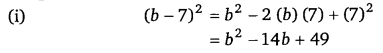Question 4.
Simplify :
(i)
(a2 – b2)2
(ii) (2x + 5)2 – (2x – 5)2
(iii) (7m – 8n)2 + (7m + 8n)2
(iv) (4m + 5n)2 + (5m + 4n)2
(v) (2.5p – 1.5q)2 – (1.5p – 2.5q)2
(vi) (ab + bc)2 – 2ab2c
(vii) (m2 – n2m)2 + 2m3n2

Solution: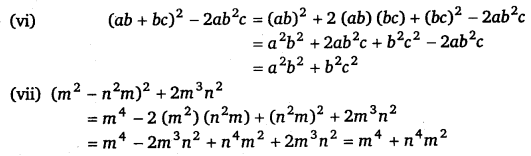Question 5.
Show that :
(i)
(3x + 7)2 – 84x = (3x – 7)2
(ii) (9p – 5q)2 + 180pq = (9p + 5q)2
(iii)$\left( \frac { 4 }{ 3 } m-\frac { 3 }{ 4 } n \right) ^{ 2 }+2mn=\frac { 16 }{ 9 } { m }^{ 2 }+\frac { 9 }{ 16 } { n }^{ 2 }$
(iv) (4pq + 3q)2 – (4pq – 3q)2 = 48pq2
(v) (a – b)(a + b) + (b – c)(b + c) + (c – a)(c + a) = 0

Solution:Question 6.
Using identities, evaluate : .
(i)
712
(ii) 992
(iii) 1022
(iv) 9982
(v) 5.22
(vi) 297 x 303
(vii) 78 x 82
(viii) 8.92
(ix) 1.05 x 9.5

Solution:Question 7.
Using a2 – b2 = (a + b) (a – b), find
(i)
512 – 492
(ii) (1.02)2 – (0.98)2
(iii) 1532 – 1472
(iv) 12.12 – 7.92

Solution: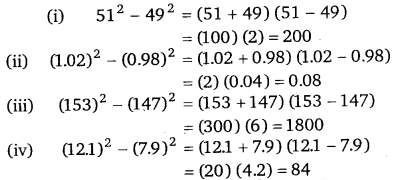Question 8.
Using (x + a) (x + b) = x + (a + b)x + ab, find
(i)
103 x 104
(ii) 5.1 x 5.2
(iii) 103 x 98
(iv) 9.7 x 9.8

Solution: